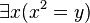Feeds:
Posts

# Sentence (mathematical logic)

This article is a technical mathematical article in the area of predicate logic. For the ordinary English language meaning see Sentence, for a less technical introductory article see Statement (logic).

In mathematical logic, a sentence of a predicate logic is a well formed formula with no free variables. A sentence is viewed by some as expressing a proposition. It makes an assertion, potentially concerning any structure of L. This assertion has a fixed truth value with respect to the structure. In contrast, the truth value of a formula (with free variables) may be indeterminate with respect to any structure. As the free variables of a formula can range over several values (which could be members of a universe, relations or functions), its truth value may vary.

##  Example

The following example is in first-order logic.is a sentence. This sentence is true in the positive real numbers but false in the real numbers. (In plain English, this sentence is interpreted to mean that every member of the structure concerned is the square of a member of that particular structure.) On the other hand, the formulais not a sentence, because of the presence of the free variable y. In the structure of the real numbers, this formula is true if we substitute y = 2, but is false if y = –2.